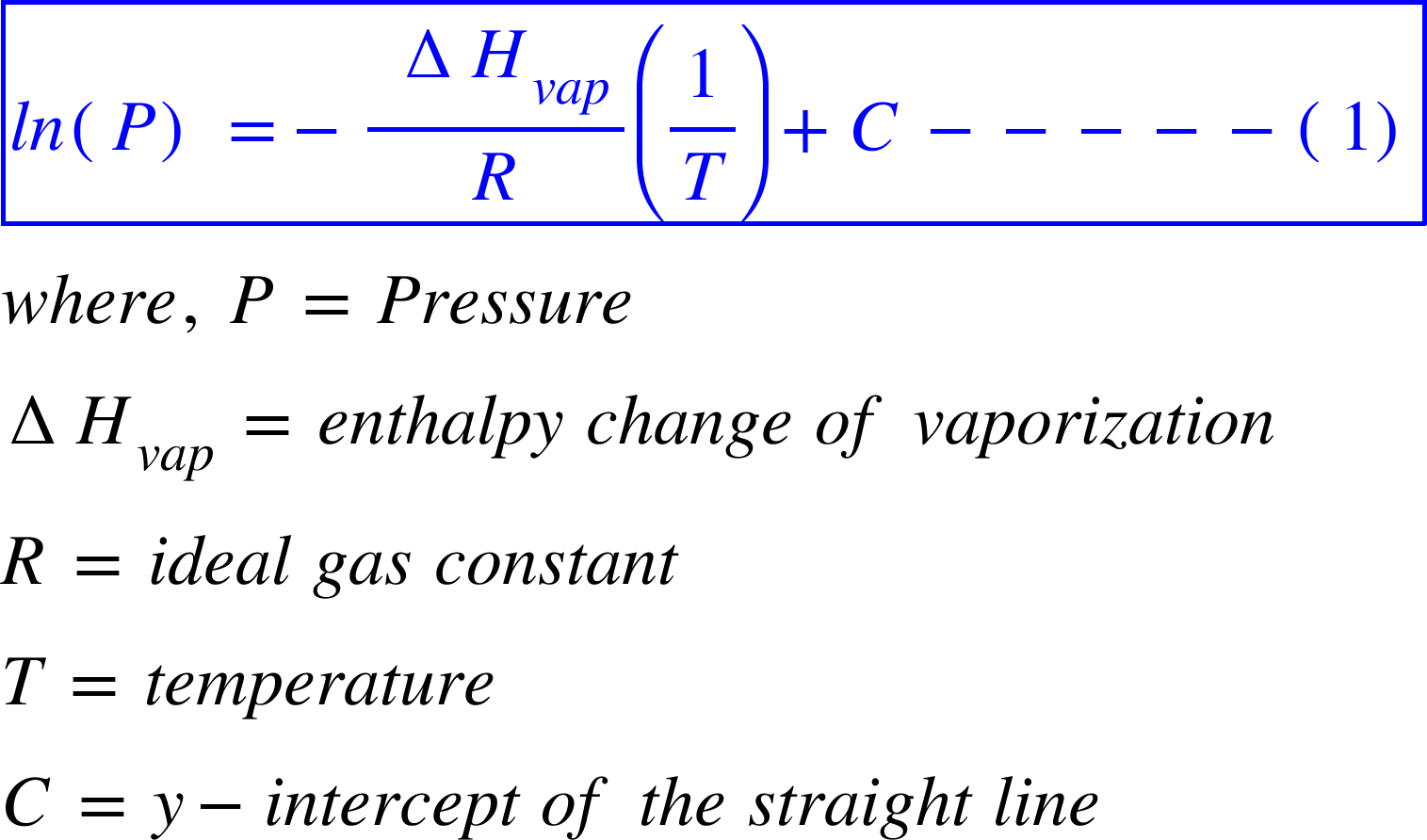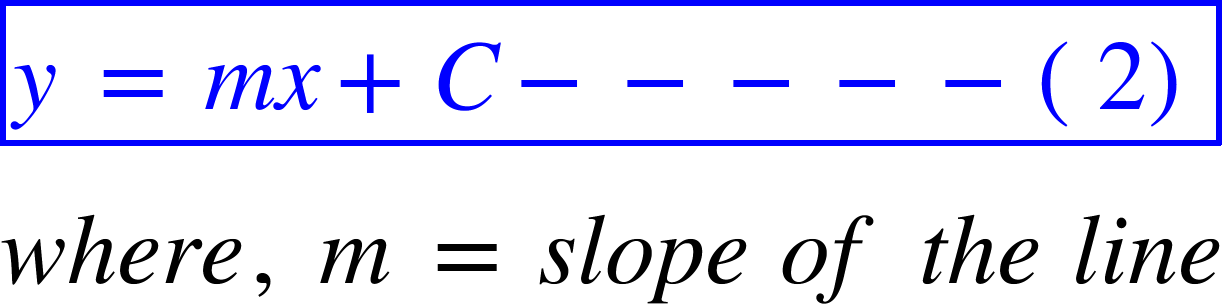# Problem: The vapor pressure for pure water and pure acetone is measured as a function of temperature. In each case, a graph of the log of the vapor pressure versus 1/T is found to be a straight line. The slope of the line for water is -4895 K and the slope of the line for acetone is -3765 K. What is the ΔHvap of water?

###### FREE Expert Solution

The vapor pressure of a liquid rises exponentially with the increase in temperature. This relation is simplified if ln(P) is plotted against 1/T and the graph becomes a straight line. The equation for such a plot is:Now, recall that the equation for a straight line is:83% (263 ratings)###### Problem Details

The vapor pressure for pure water and pure acetone is measured as a function of temperature. In each case, a graph of the log of the vapor pressure versus 1/T is found to be a straight line. The slope of the line for water is -4895 K and the slope of the line for acetone is -3765 K. What is the ΔHvap of water?